# NCERT Solutions for Class 9 Maths Exercise 1.2## myCBSEguide App

CBSE, NCERT, JEE Main, NEET-UG, NDA, Exam Papers, Question Bank, NCERT Solutions, Exemplars, Revision Notes, Free Videos, MCQ Tests & more.

NCERT solutions for Class 9 Maths Number Systems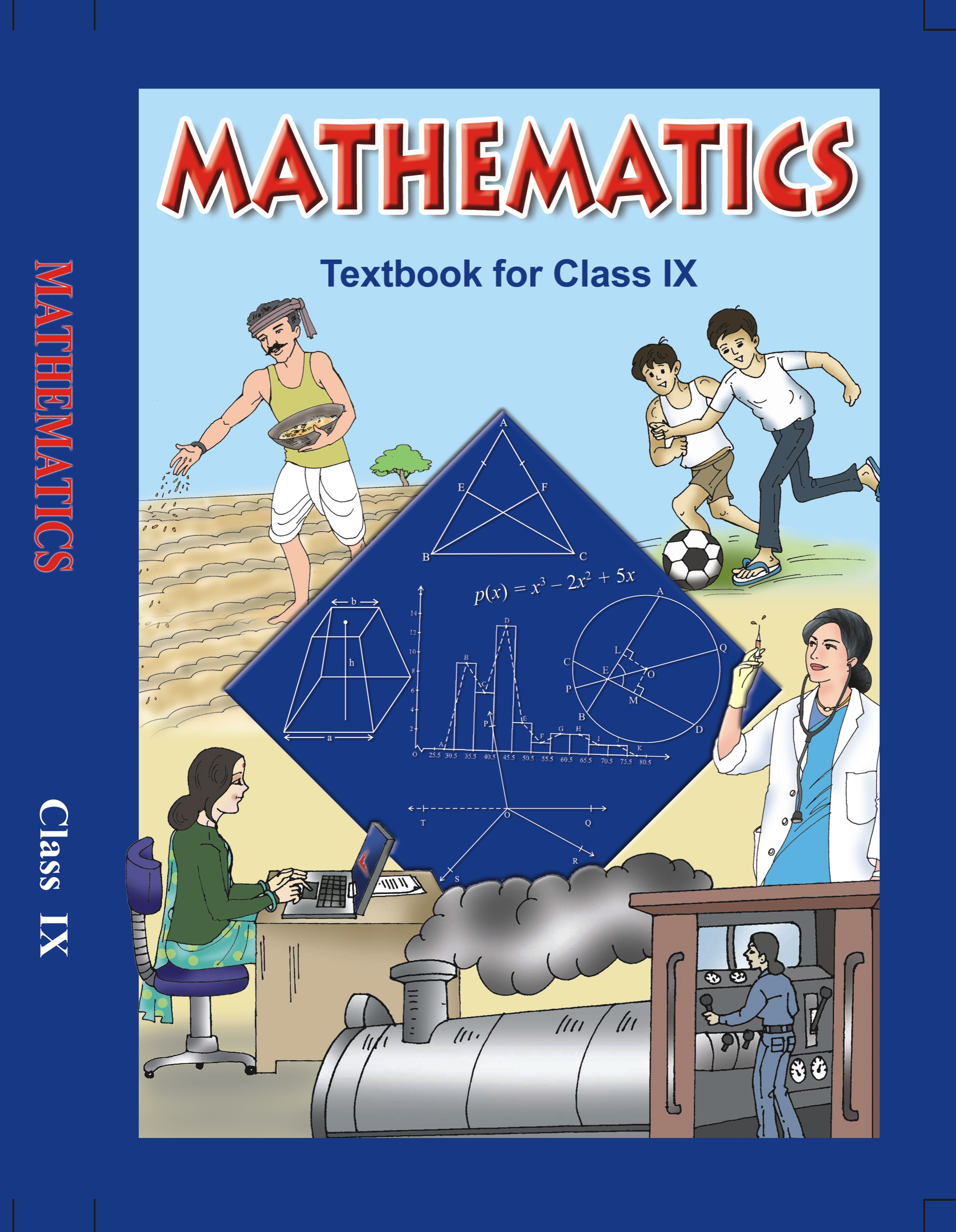## NCERT Solutions for Class 9 Maths Number Systems

###### (iii) Every real number is an irrational number.

Ans. (i) Consider the irrational numbers and the real numbers separately.

We know that irrational numbers are the numbers that cannot be converted in the form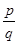, where p and q are integers and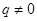.

We know that a real number is the collection of rational numbers and irrational numbers.

Therefore, we conclude that, yes every irrational number is a real number.

(ii) Consider a number line. We know that on a number line, we can represent negative as well as positive numbers.

We know that we cannot get a negative number after taking square root of any number.

Therefore, we conclude that not every number point on the number line is of the form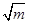, where m is a natural number.

(iii) Consider the irrational numbers and the real numbers separately.

We know that irrational numbers are the numbers that cannot be converted in the form, where p and q are integers and.

We know that a real number is the collection of rational numbers and irrational numbers.

So, we can conclude that every irrational number is a real number. But every real number is not an irrational number.

Therefore, we conclude that, every real number is not a rational number.

NCERT Solutions for Class 9 Maths Exercise 1.2

###### 2. Are the square roots of all positive integers irrational? If not, give an example of the square root of a number that is a rational number.

Ans. We know that square root of every positive integer will not yield an integer.

We know that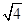is 2, which is an integer. But,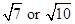will give an irrational number.

Therefore, we conclude that square root of every positive integer is not an irrational number.

NCERT Solutions for Class 9 Maths Exercise 1.2

###### 3. Show how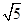can be represented on the number line.

Ans. According to the Pythagoras theorem, we can conclude that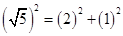.

We need to draw a line segment AB of 1 unit on the number line. Then draw a straight line segment BC of 2 units. Then join the points C and A, to form a line segment BC.

Then draw the arc ACD, to get the numberon the number line.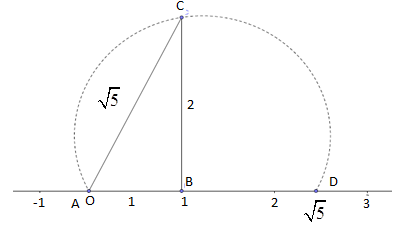## NCERT Solutions for Class 9 Maths Exercise 1.2

NCERT Solutions Class 9 Maths PDF (Download) Free from myCBSEguide app and myCBSEguide website. Ncert solution class 9 Maths includes text book solutions from Mathematics Book. NCERT Solutions for CBSE Class 9 Maths have total 15 chapters. 9 Maths NCERT Solutions in PDF for free Download on our website. Ncert Maths class 9 solutions PDF and Maths ncert class 9 PDF solutions with latest modifications and as per the latest CBSE syllabus are only available in myCBSEguide.

## CBSE app for Class 9

To download NCERT Solutions for Class 9 Maths, Computer Science, Home Science,Hindi ,English, Social Science do check myCBSEguide app or website. myCBSEguide provides sample papers with solution, test papers for chapter-wise practice, NCERT solutions, NCERT Exemplar solutions, quick revision notes for ready reference, CBSE guess papers and CBSE important question papers. Sample Paper all are made available through the best app for CBSE students and myCBSEguide website.### 3 thoughts on “NCERT Solutions for Class 9 Maths Exercise 1.2”

1. Ex 1.2 Q 1 (ii) – Negative number can be of square root of any natural number. E.g. root of 4 = +2 & -2. So your justification is wrong. Pls check and clarify

2. Steps likhane hote hai sir…

3. Very good site